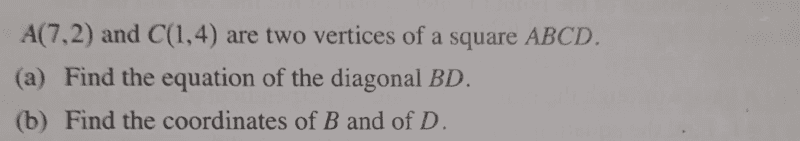# Find the equation of the straight line

• chwala

#### chwala

Gold Member
Homework Statement
See attached
Relevant Equations
straight line equations
Find the question here ( this one is a pretty easy question).I have attempted this in the past using varied approach, this is in reference to part(b) of the question... i have previously used pythagoras theorem to finding co-ordinates of ##B## and ##D##...

Anyway find my current approach on this,

The gradient of the line ##AC=\frac{-1}{3}##, it follows that the gradient of line ##BD=3##, with mid-point of ##BD##=##(4,3)##.
For part (a),
The equation of line ##BD##, is given by
##y=mx+c##
##3=12+c##
##c=-9##,
Therefore, ##y=3x-9##

For part (b),
Let the co-ordinates of ##B##=##(x_1,y_1)##
##D##=##(x_2,y_2)##
then it follows that (using gradient),
$$\frac {3}{1}=\frac {3-y_2}{4-x_2}$$
$$⇒D(x_2,y_2)=(3,0)$$
Also,
$$\frac {3}{1}=\frac {y_1-3}{x_1-4}$$
$$⇒B(x_1,y_1)=(5,6)$$

Any other way for part (2) only. Cheers

Last edited:

AB is perpendicular to BC.

C and D are on the circle centered at (4,3) with radius ##\sqrt{10}##.

•chwala
$$(x-4)^2+(y-3)^2=10$$
$$(x-4)^2+(3x-12)^2=10$$
$$x^2-8x+15=0$$
##x_1=5,⇒y_1=15-9=6##
##x_2=3⇒y_2=9-9=0##

Bingo! anutta...

The equation of the former of #2 is
$$(x-7)(x-1)+(y-2)(y-4)=0$$
which coincides with the equation of the circle.

•chwala
The equation of the former of #2 is
$$(x-7)(x-1)+(y-2)(y-4)=0$$
which coincides with the equation of the circle.
Agreed, i just used your approach in post ##3## unless you want me to show all steps. Its clear mate.